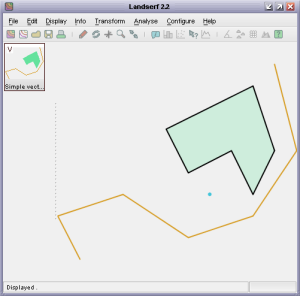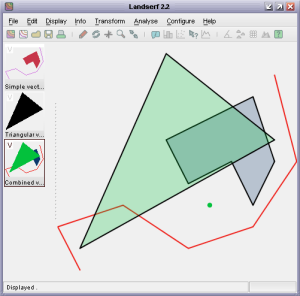This is an old version of LandSerf. For the latest release please visit www.landserf.org

# Chapter 3. Manipulating Vector Maps

By the end of this chapter you will be able to:

• Understand the relationship between a `VectorMap` and `GISVector`.

• Create `GISVector`s from sets of geometric coordinates.

• Create a vector map, add `GISVector`s to it and associate it with metadata

• Perform simple processing operations on vector maps

## 3.1 The structure of a vector map

In contrast with raster maps, vector maps are used to represent collections discrete spatial objects with known boundaries. Those objects may be points in space, lines, or areas defined by linear boundaries. A vector map may contain any number of these objects that may be separate from each other, joined, or overlapping.Simple point, line and area features and their vector map representation.

An individual vector object (such as the red line, blue line, yellow or green rectangle or point above) is represented by the class `GISVector`. This stores the geometry of the object as a series of (x,y) coordinate pairs. Implicit in this model is that lines and area boundaries are defined by joining consecutive pairs with straight line segments. A `GISVector` also inherits `SpatialObject` just as the `RasterMap` class does. It therefore has methods for defining its bounds, performing simple spatial comparisons, and manipulating its attribute(s). The `VectorMap` class simply assembles a collection of the `GISVector`s into a single object that itself has bounds and other metadata attached to it.

## 3.2 Creating a simple vector map

Creating vector maps is a simple process and just requires calling one of `VectorMap`'s constructors. Like the `RasterMap` class, you can define the bounds of the object at the point of creation, copy the contents of an existing spatial object, or simply create an empty vector map. Unlike rasters, it is more common for vector maps to grow and shrink as objects are added and removed, so it is quite usual initially to create an empty vector map object:

`VectorMap myVectorMap = new VectorMap();`

The geometry of an individual object within a vector map is stored in a `GISVector` object. The object also stores its type - one of `GISVector.POINT`, `GISVector.LINE` or `GISVector.AREA`, as well as its attribute value. These can all be set in `GISVector`'s constructor, or added later using an appropriate method.

The class below shows how some simple vector objects may be created and added to a vector map which is then saved to disk.

```import jwo.landserf.structure.*;   // For spatial object class.
import jwo.landserf.process.io.*;  // For file handling.

// **********************************************************
/** Creates a simple vector map containing some GISVectors.
* Demonstrates how vector maps can be created and written.
* @author Jo Wood
* @version 1.0, 2nd November, 2004
*/
// **********************************************************

public class CreateSimpleVector
{
//------------------ Starter Method -----------------

public static void main(String[] args)
{
new CreateSimpleVector();
}

//------------------- Constructor -------------------

public CreateSimpleVector()
{
// Create an empty vector map, then place some GISVectors within it.
VectorMap vectorMap = new VectorMap();

float riverX[] = {2,1,4,7,10,12,11};
float riverY[] = {2,4,5,3, 4, 7,11};

float buildingX[] = {6,10,11,10,9,7};
float buildingY[] = {8,10, 7, 5,7,6};

GISVector building = new GISVector(buildingX,buildingY,GISVector.AREA,20);

GISVector pump = new GISVector(8,5,30);

header.setNotes("Simple vector map containing a river, building and pump location.");

// Find range of values and create a random colour table.
float min = vectorMap.getMinAttribute();
float max = vectorMap.getMaxAttribute();
vectorMap.setColourTable(ColourTable.getPresetColourTable(ColourTable.RANDOM,min,max));

// Write new vector map to file.
LandSerfIO.write(vectorMap,"simple.vec");
}
}```

Note the similarity with the way in which raster map metadata are added and saved to disk. The vector map that is created as a result of this class is shown below.VectorMap output from the CreateSimpleVector class.

## 3.3 Manipulating Vector Maps

Vector maps can be read much as raster maps using static method `LandSerfIO.readVector(fileName)`. Altering the metadata associated with a vector map is identical to that of a raster. To manipulate an individual `GISVector` within a vector map, the set of `GISVector`s contained can be iteratively extracted using the method `getGISVectors()`. This method (slightly unfortunately for naming purposes) returns a Java `Vector` of `GISVector`s. To process each object in turn, it would be normal to create an iterator:

``` Iterator i = myVectorMap.getGISVectors().iterator();

while (i.hasNext())
{
GISVector gisVect = (GISVector)i.next();

// Do something with each GISVector here.
}
```

Depending on what you wish to do, it is sometime possible to avoid manipulating GISVector objects directly by calling appropriate methods from the `VectorMap` object. The example below shows how two vector maps can be combined with simple `clone()` and `add()` methods.

```import jwo.landserf.structure.*;   // For spatial object class.
import jwo.landserf.process.io.*;  // For file handling.

// **********************************************************
/** Combines two vector maps by overlaying one on the other
* and creating new objects from their union.
* Demonstrates how the GISVector maps can be manipulated.
* @author Jo Wood
* @version 1.1, 11th November, 2004
*/
// **********************************************************

public class CombineVectors
{
//------------------ Starter Method -----------------

public static void main(String[] args)
{
new CombineVectors();
}

//------------------- Constructor -------------------

public CombineVectors()
{
// Open an existing vector map.

// Create a simple vector map to overlay over the first one.
VectorMap vectorMap2 = new VectorMap();

float triangleX[] = {6,11,2};
float triangleY[] = {12,8,3};

GISVector triangle = new GISVector(triangleX,triangleY,GISVector.AREA,30);

header.setNotes("Simple vector map containing a triangular GISVector.");

// Clone the first vector map and add the second to it.
VectorMap vectorMap3 = (VectorMap)vectorMap1.clone();

float min = vectorMap3.getMinAttribute();
float max = vectorMap3.getMaxAttribute();
vectorMap3.setColourTable(ColourTable.getPresetColourTable(ColourTable.RANDOM,min,max));

// Write new vector map to file.
LandSerfIO.write(vectorMap3,"overlay.vec");
}
}```

The output from this class is shown in LandSerf below:VectorMap output from the CombineVectors class.

### 3.4 Exercises

1. Write a class that reads in a vector map and separates the map into 'layers' of vector maps, each contining objects with the same attribute. To do this, you will need to iterate through each `GISVector` contained within the input map, identify its attribute, and then create a new vector map or add it to an existing one.

2. Modify the `CombineVectors` class shown above so that it uses the existing colour tables of the combined vector maps (rather than creating a random one as above). You will have to consider what to do if the two vector maps have different colours assigned to the same attribute value.